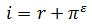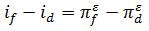### Why should I choose AnalystNotes?

AnalystNotes specializes in helping candidates pass. Period.

##### Subject 3. A Long-Term Framework for Exchange Rates
Now we begin to examine determinants of exchange rates, starting with long-term interrelationships among exchange rates, interest rates, and inflation rates. Despite their empirical shortcomings, these parity conditions form the key building blocks for many long-run exchange rate models.

Covered Interest Rate Parity

This is the only parity that holds in short and medium term. It results from riskless arbitrage and must be true at any point of time.

Uncovered Interest Rate Parity

Uncovered interest rate parity refers to the idea that the exchange rate exposure is NOT covered, hedged or protected by a forward contract. It states that the expected movement in the exchange rate should offset the interest rate differential.Therefore, the current forward exchange rate is an unbiased (i.e., correct on average) predictor of the future spot rate.

Example

If the one year interest rate for Japanese yen is 3% and 5% for U.S. dollars, and the exchange rate is \$0.00945 per yen, what is the expected future exchange rate in one year?

Δ(i) = 0.05 - 0.03 = 0.02. => This means that the spot rate is expected to increase by 2% => E(S) = 0.00945 x 1.02 = \$0.009639.

Uncovered interest rate parity is an economic theory only. In reality the future exchange rate is uncertain, and arbitrage cannot be instantaneous and costless.

Purchase Power Parity

The law of one price states that the same good cannot sell for different prices in different locations at the same time. This results in exchange-adjusted prices of identical goods and financial assets. Purchasing power parity (PPP) is an application of this principle in the international market place. It links spot exchange rates and inflation by the no arbitrage condition. In contrast, covered interest rate parity links spot rates, forward rates, and interest rates by the no arbitrage condition.

There are several versions of PPP:

• Absolute PPP states that exchange rates should equal the ratio of the average price levels of the two economies being compared.
• Relative PPP claims the exchange rate movements should exactly offset any inflation differential between the two countries.• The ex ante version of PPP states that expected changes in exchange rates should equal the difference in expected national inflation rates.The nominal exchange rate simply states how much of one currency can be traded for a unit of another currency. The real exchange rate, on the other hand, describes how many of a good or service in one country can be traded for one of that good or service in another country. For example, a real exchange rate might state how many European bottles of wine can be exchanged for one U.S. bottle of wine.

In the long run, real exchange rates tend to stabilize around their average values.

The Fisher Effect and Real Interest Rate Parity

The domestic Fisher effect states that nominal interest rates consist of a real return and the expected inflation premium.Arbitrage causes real returns to equalize across countries. As real interest rates are stable over time, and fluctuations in interest rates are caused by revisions in inflationary expectations only, the real interest rates are equal across the world. The international Fisher effect states that the interest rate differential between two countries should be equal to the expected inflation rate differential over the term of interest rate.The international Fisher effect states that countries with high inflation rates will have higher interest rates than countries with lower inflation rates.

Combining the Relations

The parity relationships are all linked with each other:

• Covered interest rate parity: The forward discount or premium is equal to the nominal interest rate differential.
• Uncovered interest rate parity: The expected currency appreciation/depreciation offsets the nominal interest rate differential.
• Purchasing power parity: Exchange rate movements are supposed to exactly offset inflation differentials.
• International Fisher relation: The expected inflation rate differential is matched by the nominal interest rate differential.

Assessing an Exchange Rates' Equilibrium Level

PPP may be used to assess the long-run equilibrium level of exchange rates. There are other approaches as well.

• The macroeconomic balance approach focuses on a country's normal current account balance.
• The external sustainability approach focuses on a country's net external asset or liability position.
• A reduced-form econometric model uses several key macroeconomic variables to estimate the long-run fair value in the fx market.